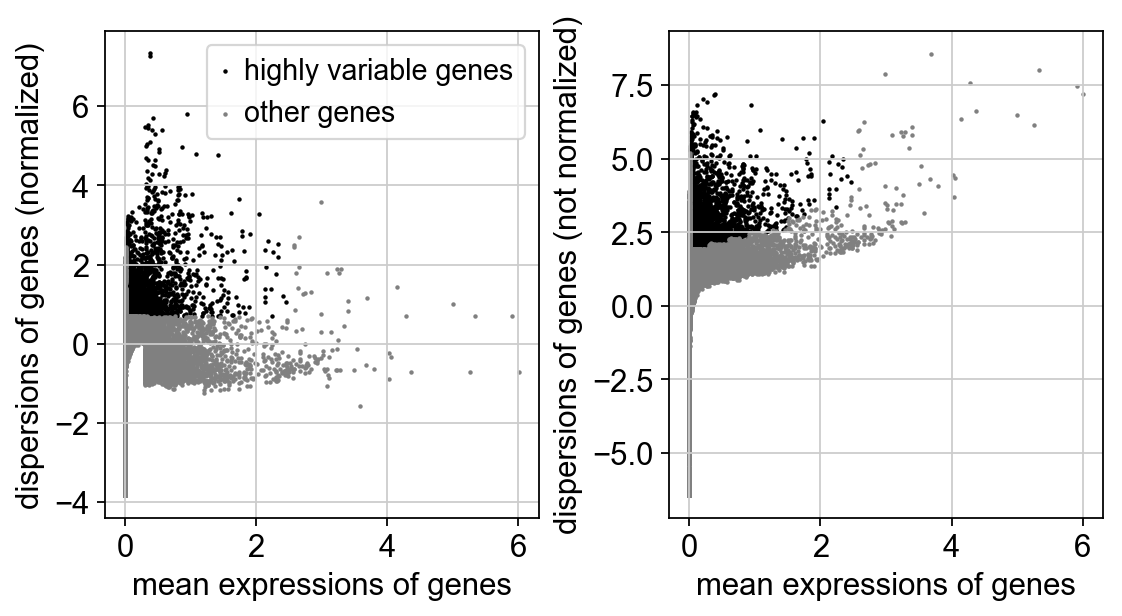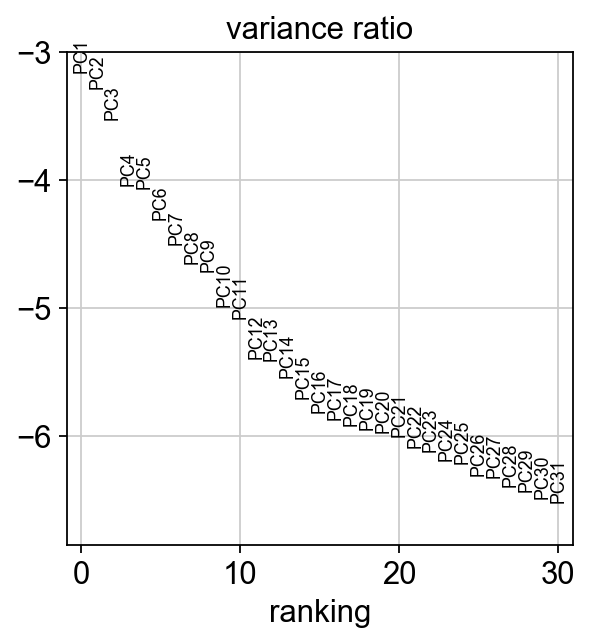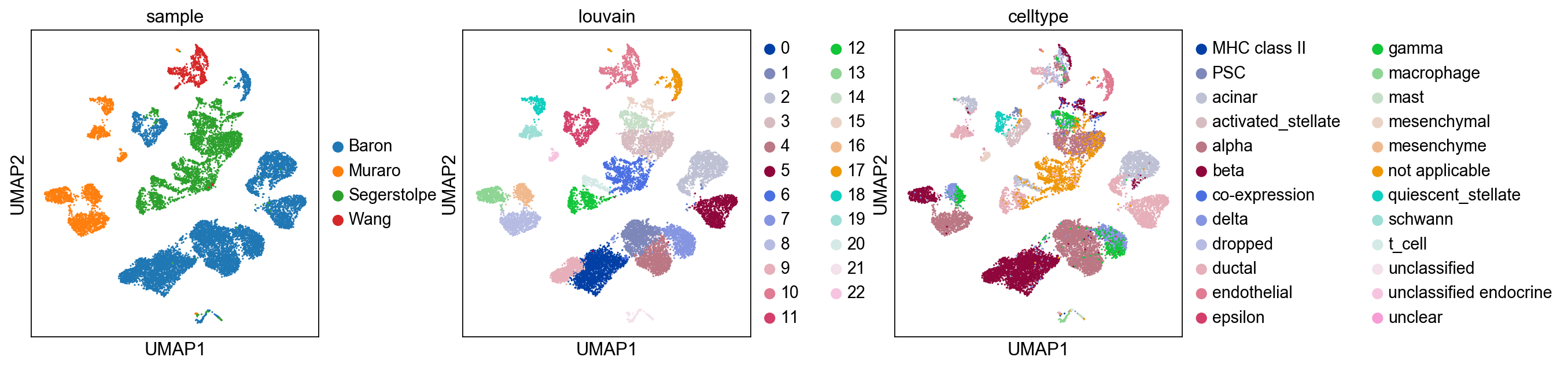In :
import numpy as np
import pandas as pd
import scanpy.api as sc
import anndata
import bbknn
import os
from scipy import sparse

sc.settings.verbosity = 3  # verbosity: errors (0), warnings (1), info (2), hints (3)
sc.settings.set_figure_params(dpi=80)  # low dpi (dots per inch) yields small inline figures
sc.logging.print_versions()

/Users/kp9/anaconda3/envs/orig/lib/python3.5/site-packages/h5py/__init__.py:36: FutureWarning: Conversion of the second argument of issubdtype from float to np.floating is deprecated. In future, it will be treated as np.float64 == np.dtype(float).type.
from ._conv import register_converters as _register_converters

scanpy==1.2.2 anndata==0.6.4 numpy==1.14.2 scipy==1.0.1 pandas==0.22.0 scikit-learn==0.19.1 statsmodels==0.8.0 python-igraph==0.7.1 louvain==0.6.0


In this notebook, we'll apply BBKNN to four pancreas datasets generated with different technologies. We'll also take a look at how trimming and data scaling impact the results. The bulk of the analysis will be based on the Seurat-like scanpy tutorial, with BBKNN slotting into the sc.pp.neighbors() spot. We'll only make use of UMAP for visualisation as TSNE does not use the neighbour graph when constructing its dimensionality reduction, and that's the part of the analysis which BBKNN alters.

It should be noted that shall you choose to re-run the code, you may get slightly differing visuals, but the overall findings should still hold. If you wish to explore the objects in their current form, they can be downloaded from ftp://ngs.sanger.ac.uk/production/teichmann/BBKNN/objects-pancreas.zip

Download the pancreas data, in the form of SCESet objects compiled by Vlad Kiselev. This ensures compatible annotations between all the datasets with minimal risk of user error.

In :
if not os.path.exists('rawdata-pancreas'):
os.makedirs('rawdata-pancreas')
os.system('wget https://scrnaseq-public-datasets.s3.amazonaws.com/scater-objects/baron-human.rds')
os.system('wget https://scrnaseq-public-datasets.s3.amazonaws.com/scater-objects/muraro.rds')
os.system('wget https://scrnaseq-public-datasets.s3.amazonaws.com/scater-objects/segerstolpe.rds')
os.system('wget https://scrnaseq-public-datasets.s3.amazonaws.com/scater-objects/wang.rds')
os.system('mv *.rds rawdata-pancreas')


SCESet is an R thing. Thankfully, there is a cool little bit of jupyter notebook wizardry known as Rmagic that allows you to run R from within a python notebook. This is installed via the package rpy2.

Be sure to check that whatever R is hooked up to rpy2 actually has SingleCellExperiment - for example, if conda'ing rpy2 in, conda fetches its own R which will not see your installed packages. In case you want to actually get into that R from outside the jupyter notebook, call R.home() from within Rmagic and add /bin/R to the path that comes up.

In :
%load_ext rpy2.ipython

In :
%%R

if (!('SingleCellExperiment' %in% rownames(installed.packages())))
{
source("https://bioconductor.org/biocLite.R")
biocLite("SingleCellExperiment")
}


With that out of the way, let's get importing! Time for four rather similar blocks of code, creating R data frames for the counts and cell type annotations, followed by AnnData objects being created for them and minimal gene/read count filtering being performed. Let's start with the Baron data.

In :
%%R -o counts -o annot

suppressMessages(library(SingleCellExperiment))

counts = data.frame(counts(holder))
annot = data.frame(as.character(colData(holder)[,'cell_type1']))

In :
holder = []
holder.append(anndata.AnnData(X=counts.values).T)
holder[-1].X = sparse.csr_matrix(holder[-1].X)
holder[-1].var_names = counts.index
holder[-1].obs_names = counts.columns
holder[-1].obs['celltype'] = annot.values
holder[-1].obs['sample'] = 'Baron'
sc.pp.filter_cells(holder[-1], min_genes=200)
sc.pp.filter_genes(holder[-1], min_cells=3)


And now the Muraro data. You'll notice a slight difference in adata.var_names parsing, as the Muraro dataset features the chromosome embedded as part of the gene name and we need to remove it.

In :
%%R -o counts -o annot

suppressMessages(library(SingleCellExperiment))

counts = data.frame(normcounts(holder))
annot = data.frame(as.character(colData(holder)[,'cell_type1']))

In :
holder.append(anndata.AnnData(X=counts.values).T)
holder[-1].X = sparse.csr_matrix(holder[-1].X)
holder[-1].var_names = [item.split('__') for item in counts.index]
holder[-1].obs_names = counts.columns
holder[-1].obs['celltype'] = annot.values
holder[-1].obs['sample'] = 'Muraro'
sc.pp.filter_cells(holder[-1], min_genes=200)
sc.pp.filter_genes(holder[-1], min_cells=3)


The Segerstolpe data.

In :
%%R -o counts -o annot

suppressMessages(library(SingleCellExperiment))

counts = data.frame(counts(holder))
annot = data.frame(as.character(colData(holder)[,'cell_type1']))

In :
holder.append(anndata.AnnData(X=counts.values).T)
holder[-1].X = sparse.csr_matrix(holder[-1].X)
holder[-1].var_names = counts.index
holder[-1].obs_names = counts.columns
holder[-1].obs['celltype'] = annot.values
holder[-1].obs['sample'] = 'Segerstolpe'
sc.pp.filter_cells(holder[-1], min_genes=200)
sc.pp.filter_genes(holder[-1], min_cells=3)


And the Wang data.

In :
%%R -o counts -o annot

suppressMessages(library(SingleCellExperiment))

counts = data.frame(normcounts(holder))
annot = data.frame(as.character(colData(holder)[,'cell_type1']))

In :
holder.append(anndata.AnnData(X=counts.values).T)
holder[-1].X = sparse.csr_matrix(holder[-1].X)
holder[-1].var_names = counts.index
holder[-1].obs_names = counts.columns
holder[-1].obs['celltype'] = annot.values
holder[-1].obs['sample'] = 'Wang'
sc.pp.filter_cells(holder[-1], min_genes=200)
sc.pp.filter_genes(holder[-1], min_cells=3)


With all of the data imported as individual AnnData objects, we can merge them. Kick out any lingering ERCCs. After doing that, we can set up .raw as a precautionary measure (which we use to reconstruct the object in a more pristine form for mnnCorrect/CCA comparison purposes later!) and scale the data to 10,000 counts per cell.

In :
adata = holder.concatenate(holder[1:], join='outer')


Identify highly variable genes. The thresholds were selected to aim for ~10% of all genes as highly variable.

In :
filter_result = sc.pp.filter_genes_dispersion(
sc.pl.filter_genes_dispersion(filter_result)
print([sum([i for i in filter_result]),len(filter_result)])[2448, 24516]


Finish preprocessing the data by filtering it to the highly variable genes, log-transforming, scaling and performing PCA.

In :
adata = adata[:, filter_result.gene_subset]
adata.obsm['X_pca'] *= -1  # multiply by -1 to match SeuratUse the elbow plot to propose a conservative 20 PC cutoff. Compute the standard neighbour graph and create the UMAP. While cells of similar type cluster together, the batch effect is glaring.

In :
num_pcs = 20

#clear out the cluster plot colour map by hand, as otherwise it stays

computing neighbors
using 'X_pca' with n_pcs = 20
finished (0:00:04.49) --> added to .uns['neighbors']
computing UMAP
running Louvain clustering
using the "louvain" package of Traag (2017)
finished (0:00:02.38) --> found 23 clusters and added
'louvain', the cluster labels (adata.obs, categorical)Replace regular neighbour inference with BBKNN, setting the neighbors_within_batch parameter to match our neighbour total for the previous step when multiplied by the number of batches. BBKNN uses adata.obs['batch'] by default to split the batches, which is accurate in our case as each of our batches corresponds to one of the datasets. The copy flag is here to create separate objects for all the demonstrated BBKNN variants and can be skipped in most regular use.

The UMAP reveals the batches to be far better mixed than previously, while retaining cell type structure. It's the easiest to spot alpha (mauve) and beta (maroon) cells get joined together across the different datasets into cohesive entities, but this also applies to other cell types.

In :
adata_bbknn = bbknn.bbknn(adata, neighbors_within_batch=5, n_pcs=num_pcs, trim=0, copy=True)


computing batch balanced neighbors
finished (0:00:03.43) --> added to .uns['neighbors']